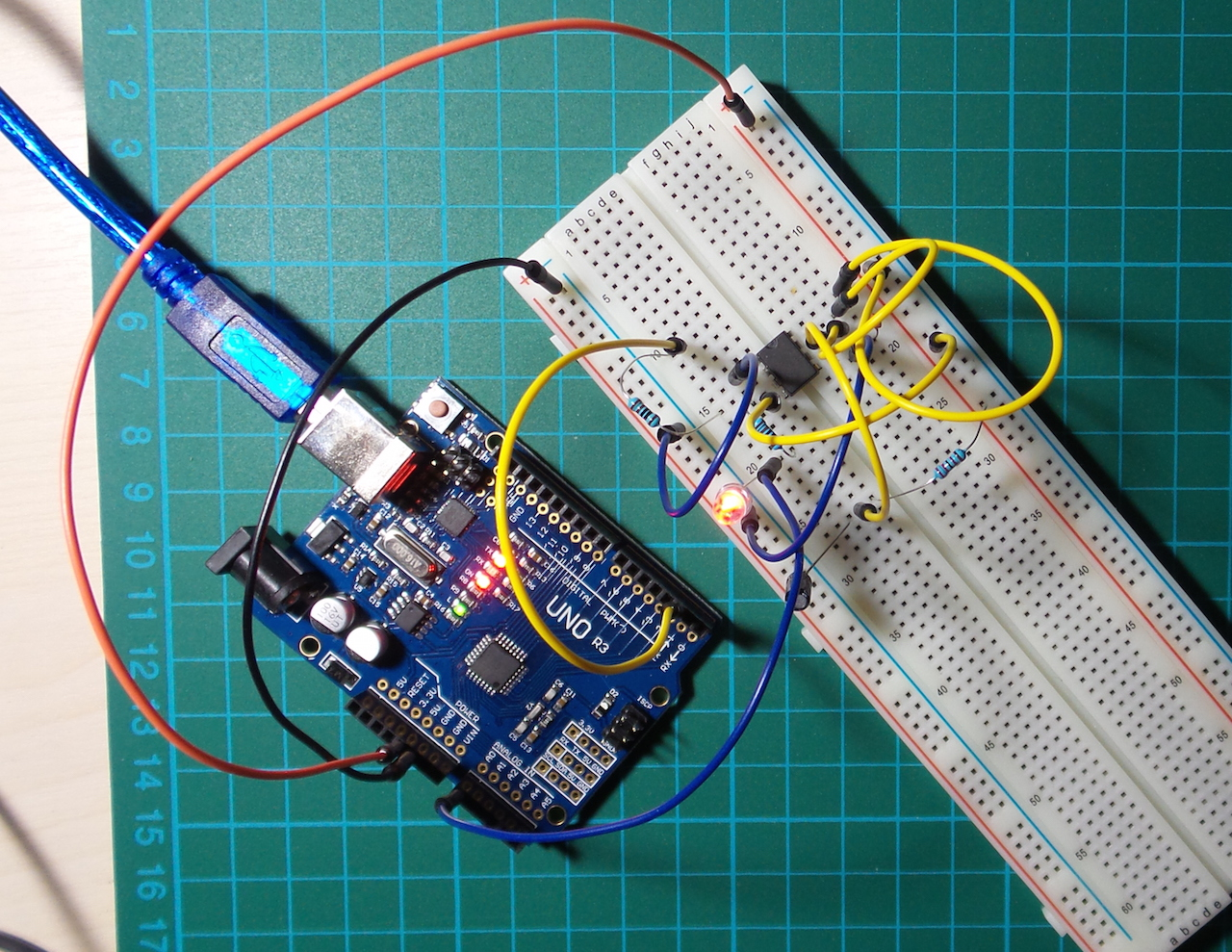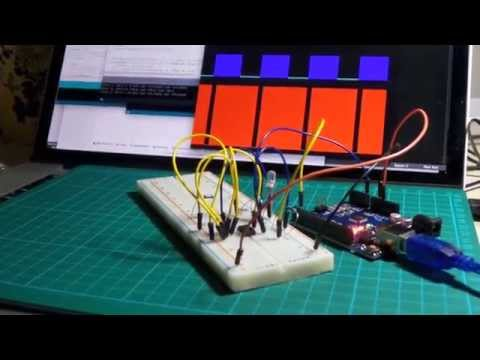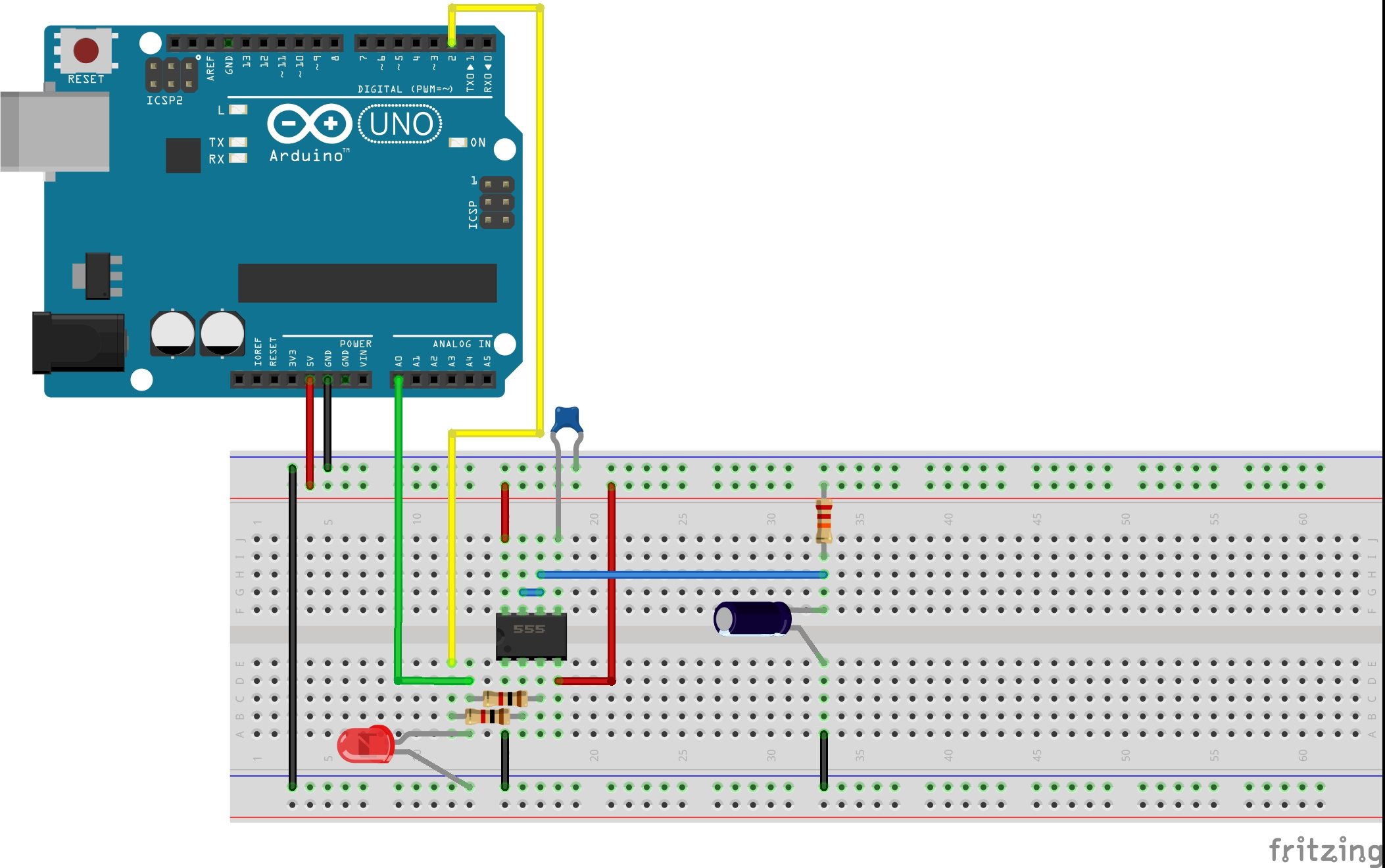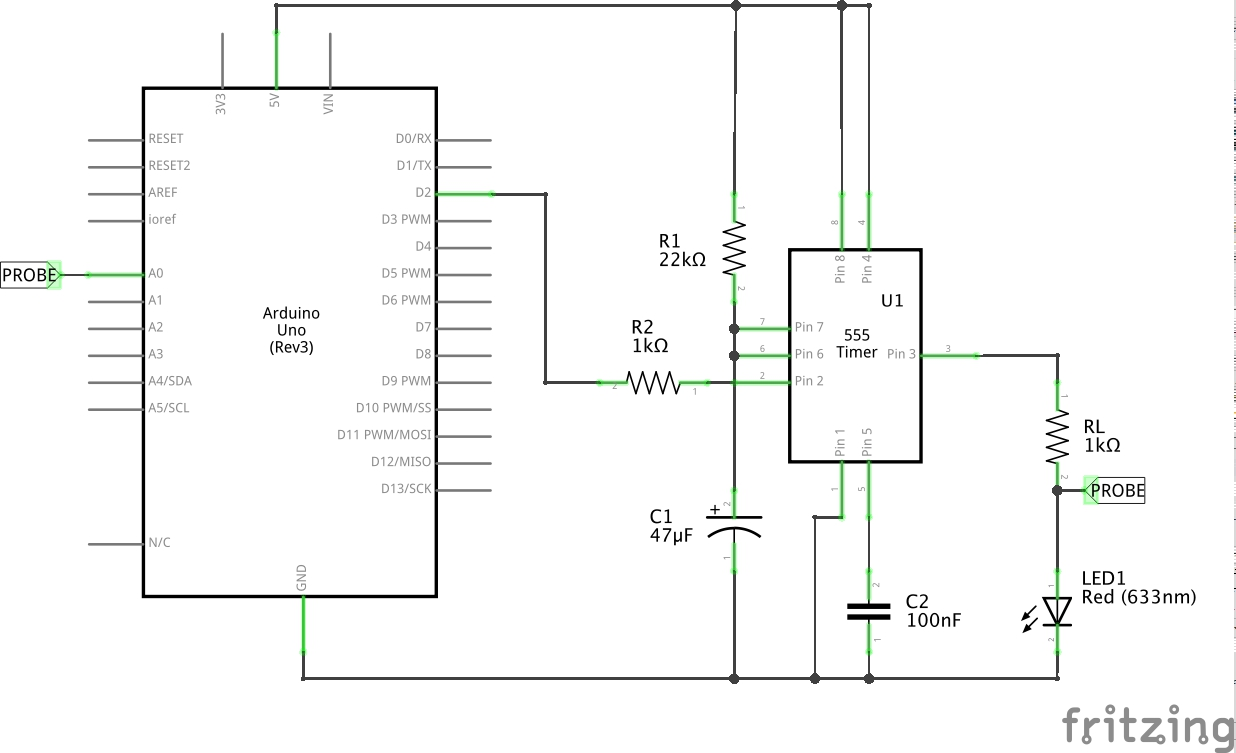# #017 555 Timer - Monostable Demo

Use an Arduino to demonstrate the monostable mode of operation, and plot the results with Processing.Here’s a quick video of the circuit in action:The monostable mode of operation is actually well described in the LM555 Datasheet.

When pin 2 is pulled low (the trigger), output pin 3 goes high and will remain high for a period of time determined by the values of R1 and C1.

### Selecting R1, C1 Values

The circuit described uses R1=22k and C1=47uF which results in the output remaining high for just over a second when triggered. The formula for calculating the high period is:

``````t = 1.1 x R1 x C1
``````

See the datasheet for your chip to confirm the formula. You can also find various websites that offer 555 timer calcuators, but I decided to write my own Visual 555 Calculator so that I could play around with HTML5 animation of the resulting circuit;-)

### Powering the project

The breadboard is powered directly from the Arduino 5V pin, although it could also be powered by an independent 5-9V supply.

### Input/Output State Plots

The output voltage is tapped at the anode of the LED, read with an analog input pin and echoed to the Arduino serial port.

PlotNValues (a simple Processing sketch) reads the data from the serial port and plots the output value over time, with some coloration effects thrown in for good measure. In other words, we’re using Arduino and Processing as a basic oscilloscope! And it kind of works, mainly because the frequency is so low.

Here’s a sample trace:### Construction